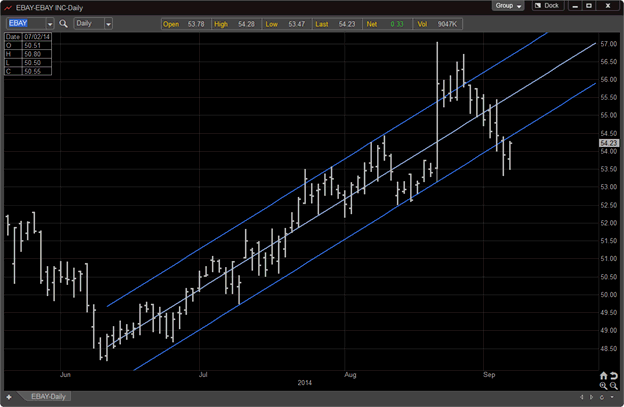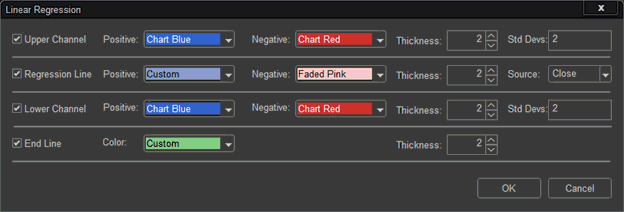# Linear RegressionLinear Regression is a drawing tool that consists of a linear regressive trend line and two parallel trend lines usually two standard deviations from the center line.

A linear regression trend line is the straight-line mathematical measurement of the relationship between sets of price data that plot out as a trend line.  It is similar to a moving average where the difference is no lag exists with a linear regression trend line.

The purpose for identifying upper and lower channels using standard deviation trend lines from a linear regression line is to identify area where statistically the market encounters greater pressure and tendencies to return closer to the mean average trend price.  The strategy is to watch for buy or sell opportunities as price swings pull toward the outer channel line extremes, or to be more prepared for a possible change-in-trend.

Linear Regression Properties

The Linear Regression allows the trader to adjust the calculations and source of the lines.  The Linear Regression can be changed based on Open, High, Low, Close, (H+L)/2, (H+L+C)/3, (O+H+L+C+)/4.

The trend lines can be turned on or off.  The thickness and color of the trend lines can be adjusted. The Linear Regression algorithm uses standard deviations of 2 for its default setting, but can be adjusted by simply typing in another number in the Std. Devs selection window.

The End Line allows the user to see where the calculation for the Linear Regression ends.